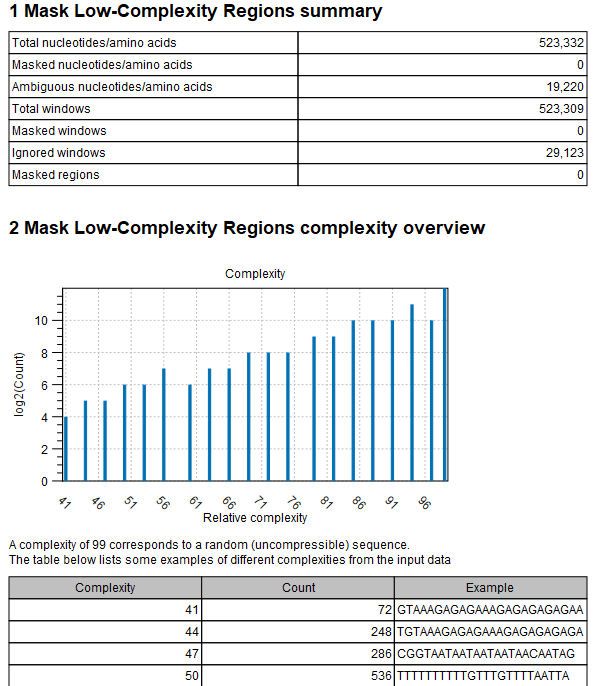An example of a Mask Low-Complexity Regions report can be seen in figure 21.5.

The summary statistics describes the following measures:

• Total nucleotides/amino acids: The total number of nucleotides (or amino acids for protein sequence input) that were processed.
• Masked nucleotides/amino acids: The total number of nucleotides (or amino acids for protein sequence input) that were masked or annotated.
• Ambiguous nucleotides/amino acids: The total number of ambiguous nucleotides (or amino acids for protein sequence input) that were masked or annotated.
• Total windows: The total number of windows that were processed (notice, that these may be overlapping)
• Masked windows: The total number of windows that were masked (notice, that these may be overlapping)
• Ignored windows: The total number of windows that were ignored (because they already contained ambiguous nucleotides, e.g. from an earlier run)
• Masked regions: The total number of regions that were masked (regions are formed by joining all overlapping or adjacent windows into contiguous sections)

The Mask Low-Complexity Regions complexity overview shows a bar chart with the number of windows for the different complexity levels.

A table provides examples from the input data for different complexity levels: this is shown in order to make it easier to understand what the Low-complexity threshold corresponds to.Figure 21.5: The Mask Low-Complexity Regions report.